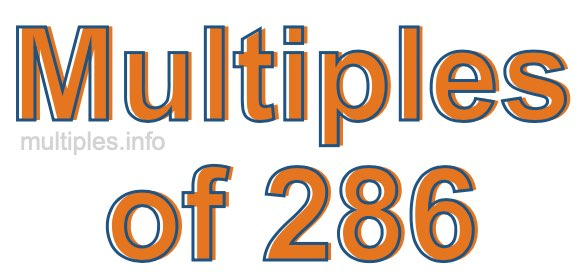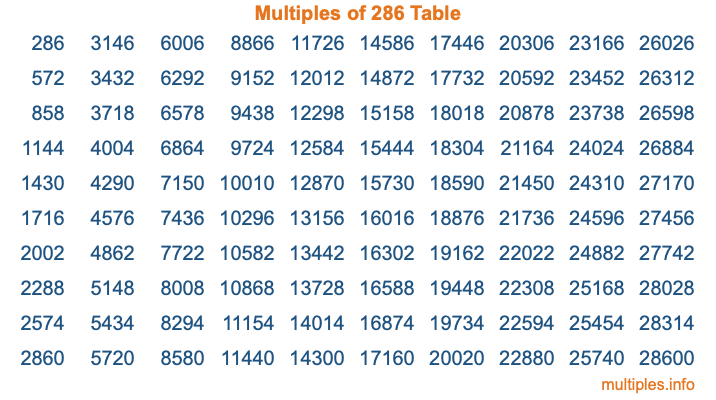Multiples of 286Welcome to the Multiples of 286 page. Here we will first teach you everything you will ever need to know about the multiples of 286, and then give you a study guide summary of everything we taught you to make sure you remember it all. Use this page to look up facts and learn information about the multiples of 286. This page will make you a multiples of two hundred eighty-six expert!

Definition of Multiples of 286
Multiples of 286 are all the numbers that when divided by 286 equal an integer. Each of the multiples of 286 are called a multiple. A multiple of 286 is created by multiplying 286 by an integer.

Therefore, to create a list of multiples of 286, you start with 1 multiplied by 286, then 2 multiplied by 286, then 3 multiplied by 286, and so on for as long as you want. Thus, the list of the first five multiples of 286 is 286, 572, 858, 1144, and 1430. To see a larger list of multiples of 286, see the printable image of Multiples of 286 further down on this page. We also have a category where you can choose any nth multiple of 286.

Multiples of 286 Checker
The Multiples of 286 Checker below checks to see if any number of your choice is a multiple of 286. In other words, it checks to see if there is any number (integer) that when multiplied by 286 will equal your number. To do that, we divide your number by 286. If the the quotient is an integer, then your number is a multiple of 286.

Is  a multiple of 286?

Least Common Multiple of 286 and ...
A Least Common Multiple (LCM) is the lowest multiple that two or more numbers have in common. This is also called the smallest common multiple or lowest common multiple and is useful to know when you are adding our subtracting fractions. Enter one or more numbers below (286 is already entered) to find the LCM.

Check out our LCM Calculator if you need more details about the Least Common Multiple or if you need the LCM for different numbers for adding and subtraction fractions.

nth Multiple of 286
As we stated above, 286 is the first multiple of 286, 572 is the second multiple of 286, 858 is the third multiple of 286, and so on. Enter a number below to find the nth multiple of 286.

th multiple of 286

Multiples of 286 vs Factors of 286
286 is a multiple of 286 and a factor of 286, but that is where the similarities end. All postive multiples of 286 are 286 or greater than 286. All positive factors of 286 are 286 or less than 286.

Below is the beginning list of multiples of 286 and the factors of 286 so you can compare:

Multiples of 286: 286, 572, 858, 1144, 1430, etc.

Factors of 286: 1, 2, 11, 13, 22, 26, 143, 286

As you can see, the multiples of 286 are all the numbers that you can divide by 286 to get a whole number. The factors of 286, on the other hand, are all the whole numbers that you can multiply by another whole number to get 286.

It's also interesting to note that if a number (x) is a factor of 286, then 286 will also be a multiple of that number (x).

Multiples of 286 vs Divisors of 286
The divisors of 286 are all the integers that 286 can be divided by evenly. Below is a list of the divisors of 286.

Divisors of 286: 1, 2, 11, 13, 22, 26, 143, 286

The interesting thing to note here is that if you take any multiple of 286 and divide it by a divisor of 286, you will see that the quotient is an integer.

Multiples of 286 Table
Below is an image of the first 100 multiples of 286 in a table. The table is in chronological order, column by column. The first column has the first ten multiples of 286, the second column has the next ten multiples of 286, and so on.The Multiples of 286 Table is also referred to as the 286 Times Table or Times Table of 286. You are welcome to print out our table for your studies.

Negative Multiples of 286
Although not often discussed or needed in math, it is worth mentioning that you can make a list of negative multiples of 286 by multiplying 286 by -1, then by -2, then by -3, and so on, to get the following list of negative multiples of 286:

-286, -572, -858, -1144, -1430, etc.

Multiples of 286 Summary
Below is a summary of important Multiples of 286 facts that we have discussed on this page. To retain the knowledge on this page, we recommend that you read through the summary and explain to yourself or a study partner why they hold true.

There are an infinite number of multiples of 286.

A multiple of 286 divided by 286 will equal a whole number.

286 divided by a factor of 286 equals a divisor of 286.

The nth multiple of 286 is n times 286.

The largest factor of 286 is equal to the first positive multiple of 286.

286 is a multiple of every factor of 286.

286 is a multiple of 286.

A multiple of 286 divided by a divisor of 286 equals an integer.

286 divided by a divisor of 286 equals a factor of 286.

Any integer times 286 will equal a multiple of 286.

Multiples of a Number
Here you can get the multiples of another number, all with the same attention to detail as we did for multiples of 286 on this page.

Multiples of
Multiples of 287
Did you find our page about multiples of two hundred eighty-six educational? Do you want more knowledge? Check out the multiples of the next number on our list!